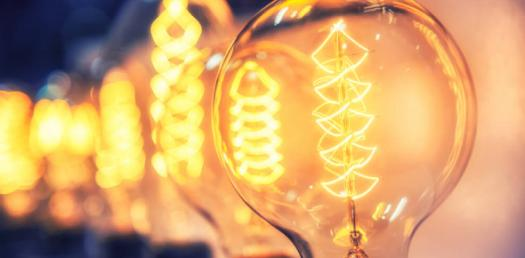# Calculation Of Electrical Energy! Trivia Questions Quiz

5 Questions | Total Attempts: 83Settings.

• 1.
How much charge flows through an appliance rated 240V, 60W when it is used for 5 mins?
• A.

75.0 C

• B.

0.25 C

• C.

40.0 C

• D.

1.25 C

• 2.
The e.m.f of a cell is 1.5V. What energy is dissipated by the cell if it drives 0.4C of charge round the circuit?
• A.

0.6 J

• B.

0.6 W

• C.

3.75 J

• D.

3.75 W

• 3.
What is the e.m.f of a cell if it uses 4.2 joules of energy as it pushes 6 coulombs of charge around a circuit?
• A.

0.7 V

• B.

25.2 V

• C.

1.43 V

• D.

4.8 V

• 4.
What is electrical energy converted as heat by a 240 V light bulb that is switched on for 300 seconds? A current of 5A is moving through the bulb.
• A.

360000 J

• B.

72000 J

• C.

1500 J

• D.

14400 J

• 5.
What is the power of a light bulb if a 240 V light bulb is switched on for 2 minutes? A current of 3 A is moving through the bulb.
• A.

720 Watts

• B.

1440 Watts

• C.

86400 Watts

• D.

840 Watts

Related TopicsBack to top